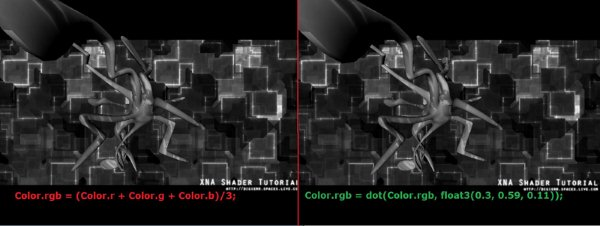### 1．平均颜色

Color.rgb = (Color.r + Color.g + Color.b)/3;

### 2．灰度强度

g = 0.3 R + 0.59 G + 0.11 B

Color.rgb = dot(Color.rgb, float3(0.3, 0.59, 0.11));### 1．平均颜色

sampler ColorMapSampler : register(s0);
float4 PixelShader(float2 Tex: TEXCOORD0) : COLOR
{
float4 Color = tex2D(ColorMapSampler, Tex);
Color.rgb = (Color.r + Color.g + Color.b)/3;

// Keep our alphachannel at 1.
Color.a = 1.0f;
return Color;
}

technique PostProcess
{
pass P0
{
}
} 

### 2. 灰度强度

sampler ColorMapSampler : register(s0);
{
float4 Color = tex2D(ColorMapSampler, Tex);
Color.rgb = dot(Color.rgb, float3(0.3, 0.59, 0.11));

// Keep our alphachannel at 1.
Color.a = 1.0f;
return Color;
}

technique PostProcess
{
pass P0
{
}